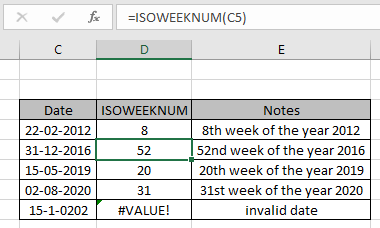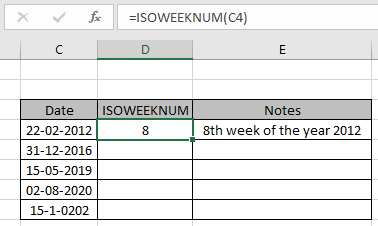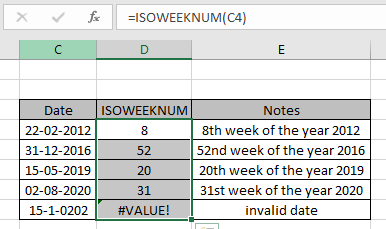# How to Use ISOWEEKNUM Function in ExcelThe Excel ISOWEEKNUM function is a DATE & TIME function. This function takes the date value of any valid year as input and returns the week number of any particular year as numerical format. In this article, we will learn how to use the ISOWEEKNUM function in Excel. DATE value in excel is stored as number.

Syntax of ISOWEEKNUM Function:

=ISOWEEKNUM(date)

date : valid date

Example:
All of these might be confusing to understand. So, let's test this formula via running it on the example shown below. So, here I have a table of some data. Excel understands date value as numerical value. We will get the week number for each of the given date values.

Use the formula:

=ISOWEEKNUM(C4)It will return 8. Here the argument to the ISOWEEKNUM function is given using cell reference. The returned value by ISOWEEKNUM function is 8 as excel calculates the date 22-02-2012 comes in the 8th week of the 2012 year. Now You can copy the formula from one to the remaining cells using the Ctrl + D shortcut key or using the drag down option in excel.As you can see from the above snapshot that ISOWEEKNUM function takes date value as input and returns the week number of the given input date as numeric value. The function returns #VALUE! Error if the date value is invalid.

Here are some observational notes shown below.

Notes:

1. The formula only works with numbers.
2. The formula returns 52 as the last week number of any year.
3. Beware to check the blank cells as the formula returns 52 for the blank cells.
4. The formula returns 52 for the value 0 as date input.
5. The function returns #VALUE! Error if the date or time value is invalid.

Hope this article about How to use the ISOWEEKNUM function in Excel is explanatory. Find more articles on DATE & TIME formulas here. If you liked our blogs, share it with your fristarts on Facebook. And also you can follow us on Twitter and Facebook. We would love to hear from you, do let us know how we can improve, complement or innovate our work and make it better for you. Write to us at info@exceltip.com.

Related Article

minutes to hours and minutes | returns a numerical value which represents the same value but in decimal format in place of time format in excel.

Calculate hours between time in Excel | Calculate the hours between two time values in excel. find the time elapsed between end_time & start_time

Time difference in hours as decimal value | Time difference in hours using Excel MOD function and then we need to represent it in the decimal format.

SUM time over 9 hours in Excel | get the sum of time values which are above 9 hours

How to add Decimal Hours to Time | add numeric or decimal time to a time formatted value.

Popular Articles:

50 Excel Shortcuts to Increase Your Productivity | Get faster at your task. These 50 shortcuts will make you work even faster on Excel.

The VLOOKUP Function in Excel | This is one of the most used and popular functions of excel that is used to lookup value from different ranges and sheets.

COUNTIF in Excel 2016 | Count values with conditions using this amazing function. You don't need to filter your data to count specific values. Countif function is essential to prepare your dashboard.

How to Use SUMIF Function in Excel | This is another dashboard essential function. This helps you sum up values on specific conditions.

Terms and Conditions of use

The applications/code on this site are distributed as is and without warranties or liability. In no event shall the owner of the copyrights, or the authors of the applications/code be liable for any loss of profit, any problems or any damage resulting from the use or evaluation of the applications/code.